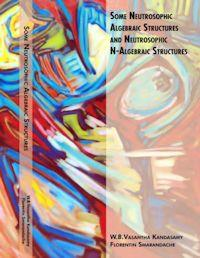#jsDisabledContent { display:none; } My Account | Register | Help

AlgebraAlgebra is the branch of mathematics concerning the study of the rules of operations and relations, and the constructions and concepts arising from them, including terms, polynomials, equations and algebraic structures. Together with geometry, analysis, topology, combinatorics, and number theory, algebra is one of the main branches of pure mathematics.•Dividing polynomials : Dividing polynomials with remainders

By: Sal Khan, Monterey Institute for Technology and Education

Dividing polynomials with remainders

Using polynomial expressions and factoring polynomials.

•Multiplying monomials, binomials and polynomials in general : Mult...

By: Sal Khan, Monterey Institute for Technology and Education

Multiplying Polynomials

Using polynomial expressions and factoring polynomials.

•Polynomial basics : Evaluating a polynomial at a given value

By: Sal Khan, Monterey Institute for Technology and Education

Evaluating a polynomial at a given value

Using polynomial expressions and factoring polynomials.

•Polynomial basics : Interesting Polynomial Coefficient Problem

By: Sal Khan

Finding the coefficients of a third degree polynomial given 2 roots and the y-intercept

Using polynomial expressions and factoring polynomials.

•Polynomial basics : Terms coefficients and exponents in a polynomial

By: Sal Khan, Monterey Institute for Technology and Education

Terms coefficients and exponents in a polynomial

Using polynomial expressions and factoring polynomials.

•Polynomial basics : Simply a polynomial

By: Sal Khan, Monterey Institute for Technology and Education

Simply a polynomial

Using polynomial expressions and factoring polynomials.

•Inverting matrices : Matrices to solve a system of equations

By: Sal Khan

Using the inverse of a matrix to solve a system of equations.

Understanding and solving matrices.

•Inverting matrices : Matrices to solve a vector combination problem

By: Sal Khan

Using matrices to figure out if some combination of 2 vectors can create a 3rd vector

Understanding and solving matrices.

•Inverting matrices : Inverting Matrices (part 3)

By: Sal Khan

Using Gauss-Jordan elimination to invert a 3x3 matrix.

Understanding and solving matrices.

•Inverting matrices : Inverting matrices (part 2)

By: Sal Khan

Inverting a 3x3 matrix

Understanding and solving matrices.

•Inverting matrices : Singular Matrices

By: Sal Khan

When and why you can't invert a matrix.

Understanding and solving matrices.

•Matrices for solving systems by elimination : Matrices: Reduced Ro...

By: Sal Khan

And another example of solving a system of linear equations by putting an augmented matrix into reduced row echelon form

Let's get our feet (and probably the rest of our body) wet in thinking in terms of vectors and spaces.

•Matrices for solving systems by elimination : Matrices: Reduced Ro...

By: Sal Khan

Solving a system of linear equations by putting an augmented matrix into reduced row echelon form

Let's get our feet (and probably the rest of our body) wet in thinking in terms of vectors and spaces.

•Matrices for solving systems by elimination : Matrices: Reduced Ro...

By: Sal Khan

Another example of solving a system of linear equations by putting an augmented matrix into reduced row echelon form

Let's get our feet (and probably the rest of our body) wet in thinking in terms of vectors and spaces.

•Natural logarithms : Calculator for Natural Logarithms

By: Sal Khan, Monterey Institute for Technology and Education

Calculator for Natural Logarithms

Log-a-what? No, this tutorial is about neither chopped wood nor music (actually logarithms do have applications in music), but it is fascinating nonetheless. You know how to take an exponent. Now you can think about what exponent you have to raise a numbe

•Some Neutrosophic Algebraic Structures and Neutrosophic N-Algebrai...

By: Florentin Smarandache; W. B. Vasantha Kandasamy

This book has seven chapters. Chapter one provides several basic notions to make this book self-contained. Chapter two introduces neutrosophic groups and neutrosophic N-groups and gives several examples. The third chapter deals with neutrosophic semigroups and neutrosophic N-semigroups, giving several interesting results. Chapter four introduces neutrosophic loops and neutrosophic N-loops. Chapter five just introduces the concept of neutrosophic groupoids and neutrosophi...

1.1 Groups, N-group and their basic Properties It is a well-known fact that groups are the only algebraic structures with a single binary operation that is mathematically so perfect that an introduction of a richer structure within it is impossible. Now we proceed on to define a group. DEFINITION 1.1.1: A non empty set of elements G is said to form a group if in G there is defined a binary operation, called the product and denoted by '•' such that

•Fuzzy Interval Matrices, Neutrosophic Interval Matrices and Their ...

By: Florentin Smarandache; W. B. Vasantha Kandasamy

The new concept of fuzzy interval matrices has been introduced in this book for the first time. The authors have not only introduced the notion of fuzzy interval matrices, interval neutrosophic matrices and fuzzy neutrosophic interval matrices but have also demonstrated some of its applications when the data under study is an unsupervised one and when several experts analyze the problem.

1.2 Definition of Fuzzy Cognitive Maps In this section we recall the notion of Fuzzy Cognitive Maps (FCMs), which was introduced by Bart Kosko in the year 1986. We also give several of its interrelated definitions. FCMs have a major role to play mainly when the data concerned is an unsupervised one. Further this method is most simple and an effective one as it can analyse the data by directed graphs and connection matrices. DEFINITION 1.2.1: An FCM is a directed graph ...

•An Elementary Treatise on Logarithms : With Tables of the Logarithms

By: Webster Wells
•Tables for the Formation of Logarithms and Anti-Logarithms to Twen...

By: Peter Gray
•Tables of Logarithms and Anti-Logarithms to Five Places, With Marginal

By: Ebenezer Erskine ScottCopyright © World Library Foundation. All rights reserved. eBooks from Hawaii eBook Library are sponsored by the World Library Foundation,
a 501c(4) Member's Support Non-Profit Organization, and is NOT affiliated with any governmental agency or department.